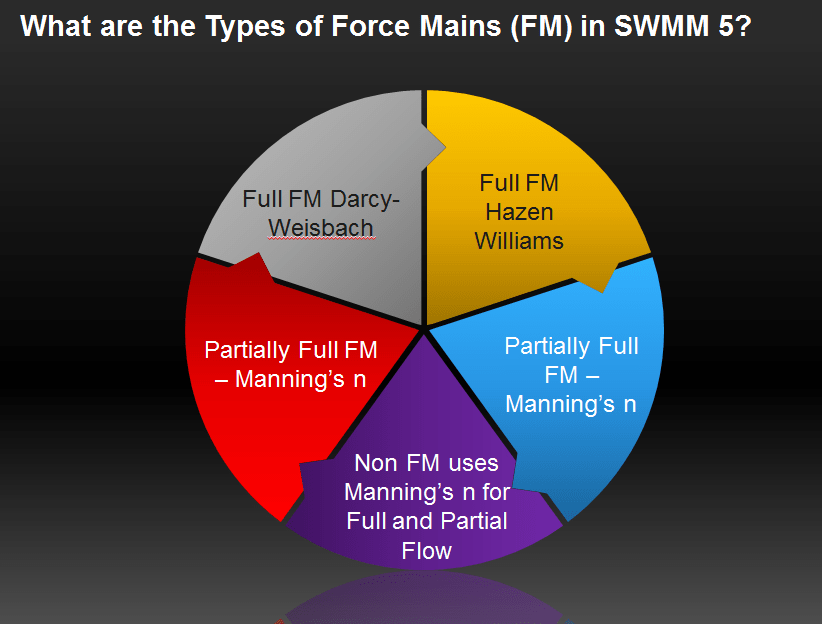#INFOSWMM

What are the Types of Force Mains (FM) in SWMM 5?

Subject:   What are the Types of Force Mains (FM) in SWMM 5?

There are five ways to model a force main in SWMM 5 for the combination of full and partial flow in the force main (Figure 1):

1.       Full Flow using Darcy-Weisbach for the friction loss

2.      Full Flow using Hazen-Williams for the friction loss

3.      Full Flow using Manning's n for the friction loss

4.      Partial Flow uses Manning's n for the friction loss for Force Main Equation options

If you use Darcy-Weisbach or Hazen-Williams then an equivalent Manning's n for a force main that results in the same normal flow value for a force main flowing full under fully turbulent conditions is calculated internally in SWMM 5 in forcemain.c

·         Equivalent n for H-W is 1.067 / Hazen-Williams Coefficient  * (Full Depth / Bed Slope) ^ 0.04

·         Equivalent n for D-W is (Darcy-Weisbach friction factor/185) * (Full Depth) ^ 1/6Figure 1.  Types of Full and Partially Full Force Mains in SWMM 5